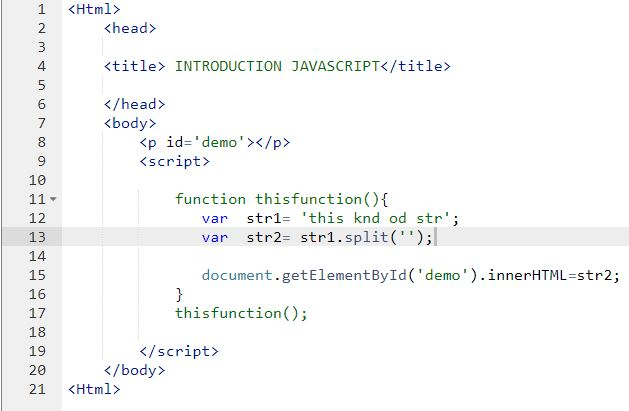## Getting Started with JavaScript

This page is for sample codes and working examples in JavaScripts using p5.js.

To learn more on how to install, setup, and prepare the environment to  writing and testing JavaScript codes, Go to this page. You can also find other  examples on that page.

In our first example, we will be writing a little script that creates bubbles.

Feel free to edit the script and improve on it and share on the page so that others can see.

A simple JavaScript code that creates bubbles on the screen.

 //***************  Create simple Bubbles in javascriptvar points =[];function setup() {    createCanvas(600, 400);    for (let i = 0; i< 100; i++ ){ var j = new Point(random(width), random(height)); points.push(j);    }} function draw(){ background(0); for (let p of points){  p.move();  p.render();   }}class Point{ constructor(x, y){  this.x = x;  this.y = y;  this.r = 8; } move(){  this.x= this.x+random(-1, 1);  this.y=this.y+random(-1, 1); } render(){  noStroke();  fill(255);  ellipse(this.x, this.y, this.r); }}